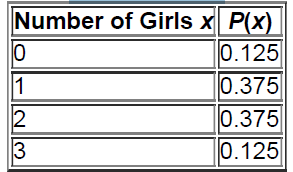×
Get Full Access to Elementary Statistics - 12 Edition - Chapter 5.2 - Problem 1bsc
Get Full Access to Elementary Statistics - 12 Edition - Chapter 5.2 - Problem 1bsc

×

# Random Variable Table 5-7 lists probabilities for theISBN: 9780321836960 18

## Solution for problem 1BSC Chapter 5.2

Elementary Statistics | 12th Edition

• Textbook Solutions
• 2901 Step-by-step solutions solved by professors and subject experts
• Get 24/7 help from StudySoup virtual teaching assistantsElementary Statistics | 12th Edition

4 5 1 388 Reviews
14
3
Problem 1BSC

Random Variable Table 5-7 lists probabilities for the corrsponding numbers of girls in three births. What is the random variable, what are its possible values, and are its values numerical?

Table 5-7 Number of Girls in Three BirthsStep-by-Step Solution:
Step 1 of 3

Solution 1BSC

From the given table, x = number of girls in three births is a random variable because its numerical values depend on chance. With three births, the number of girls can be (possible values) . 0, 1, 2, or 3. The variable x is a numerical random variable.

Step 2 of 3

Step 3 of 3

##### ISBN: 9780321836960

Unlock Textbook Solution